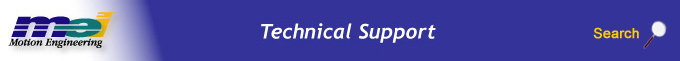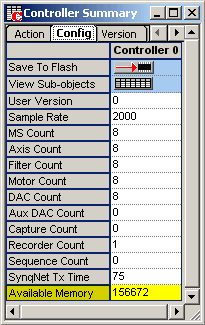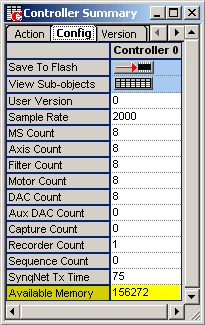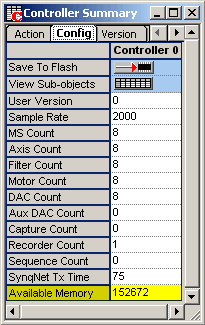.

### With this information we can now configure the size of our compensation table on the controller using MPIControlConfig.compensatorPointCount = 105.

Because the compensator table data is stored directly on the controller, it is important to know how much memory is available. This information can be found using Motion Console under the Controller Summary Window > Config Tab.

The three screenshots below show the available memory for no compensator table, compensator table with 100 points, and compensator table with 1000 points, respectively. Having 100 points requires 400 (156272 – 156672) bytes of controller memory and having 1000 points requires 4000 (156672 – 152672).

 No Compensator TableCompensator Table (size = 100)Compensator Table (size = 1000)Each data point will require 4 bytes of controller memory. In the "No Compenstor Table" example above, a maximum of 39168 (156672 / 4) points can be created.

NOTE: Although having more compensator points gives a better representation of a system; it would also significantly increase the time needed to gather all of the required data. For instance, if you added 20000 points and it took an average of 5 seconds to collect the data for that point, it would take you 100000 (20000 * 5) seconds = 1.16 days to collect that data.

 Legal Notice  |  Tech Email  |  Feedback        Copyright © 2001-2021 Motion Engineering